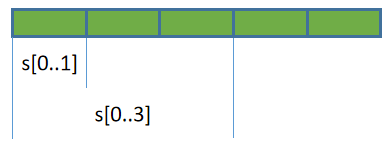## Slice类型

Slice类型通常翻译为切片，它表示从某个包含多个元素的容器中取得局部数据，这个过程称为切片操作。不同语言对切片的支持有所不同，比如有些语言只允许取得连续的局部元素，而有些语言可以取得离散元素，甚至有些语言可以对hash结构进行切片操作。

Rust也支持Slice操作，Rust中的切片操作只允许获取一段连续的局部数据，切片操作获取到的数据称为切片数据

Rust常见的数据类型中，有三种类型已支持Slice操作：String类型、Array类型和Vec类型(本文介绍的Slice类型自身也支持切片操作)。实际上，用户自定义的类型也可以支持Slice操作，只要自定义的类型满足一些条件即可，相关内容以后再介绍。

### slice操作

• `s[n1..n2]`：获取s中index=n1到index=n2(不包括n2)之间的所有元素
• `s[n1..]`：获取s中index=n1到最后一个元素之间的所有元素
• `s[..n2]`：获取s中第一个元素到index=n2(不包括n2)之间的所有元素
• `s[..]`：获取s中所有元素
• 其他表示包含范围的方式，如`s[n1..=n2]`表示取index=n1到index=n2(包括n2)之间的所有元素### slice作为数据类型

Slice类型是一个胖指针，它包含两份元数据：

• 第一份元数据是指向源数据中切片起点元素的指针
• 第二份元数据是切片数据中包含的元素数量，即切片的长度

Slice类型的描述方式为`[T]`，其中`T`为切片数据的数据类型。例如对存放了i32类型的数组进行切片，切片数据的类型为`[i32]`

``````fn main(){
let arr = [11,22,33,44,55];
let n: usize = 3;

// 编译错误，无法直接使用切片类型
let arr_s = arr[0..n];
}
``````

``````fn main(){
let mut arr = [11,22,33,44];

// 不可变slice
let arr_slice1 = &arr[..=1];
println!("{:?}", arr_slice1); // [11,22];

// 可变slice
let arr_slice2 = &mut arr[..=1];
arr_slice2 = 1111;
println!("{:?}", arr_slice2);// [1111,22];
println!("{:?}", arr);// [1111,22,33,44];
}
``````

### 特殊对待的str切片类型

``````fn main(){
let mut s = String::from("HELLO");
let ss = &mut s[..];

// make_ascii_lowercase()
// make_ascii_uppercase()
ss.make_ascii_lowercase();
println!("{}", s);  // hello
}
``````

### Array类型自动转换为Slice类型

``````#![allow(unused)]
fn main() {
let arr = [11,22,33,44];
let slice = &arr;   // &arr将自动转换为slice类型

// 调用slice类型的方法first()返回slice的第一个元素
println!("{}", slice.first().unwrap());  // 11
}
``````

``````#![allow(unused)]
fn main() {
let arr = [11, 22, 33, 44];

// 点运算符会自动将arr.first()转换为&arr.first()
// 而&arr又会自动转换为slice类型
println!("{}", arr.first().unwrap());
}
``````

### Slice类型支持的方法

Slice支持很多方法，这里介绍几个比较常用的方法，更多方法可参考官方手册：https://doc.rust-lang.org/std/primitive.slice.html#impl

• len()：取slice元素个数
• is_empty()：判断slice是否为空
• contains()：判断是否包含某个元素
• repeat()：重复slice指定次数
• reverse()：反转slice
• join()：将各元素压平(flatten)并通过指定的分隔符连接起来
• swap()：交换两个索引处的元素，如`s.swap(1,3)`
• windows()：以指定大小的窗口进行滚动迭代
• starts_with()：判断slice是否以某个slice开头

``````#![allow(unused)]
fn main() {
let arr = [11,22,33];
println!("{}",   arr.len());  // 3
println!("{:?}", arr.repeat(2)); // [11, 22, 33, 11, 22, 33]
println!("{:?}", arr.contains(&22)); // true

// reverse()
let mut arr = [11,22,33];
arr.reverse();
println!("{:?}",arr); // [33,22,11]

// join()
println!("{}", ["junma","jinlong"].join(" ")); // junma jinlong
println!("{:?}", [[1,2],[3,4]].join(&0)); // [1,2,0,3,4]

// swap()
let mut arr = [1,2,3,4];
arr.swap(1,2);
println!("{:?}", arr); // [1,3,2,4]

// windows()
let arr = [10, 20, 30, 40];
for i in arr.windows(2) {
println!("{:?}", i); // [10,20], [20,30], [30,40]
}

// starts_with()，相关的方法还有ens_with()
let arr = [10, 20, 30, 40];
println!("{}", arr.starts_with(&));  // true
println!("{}", arr.starts_with(&[10, 20])); // true
println!("{}", arr.starts_with(&));  // false
}
``````